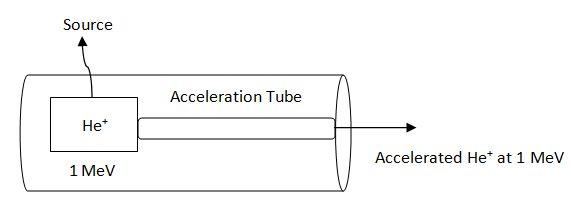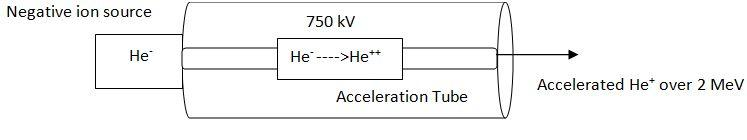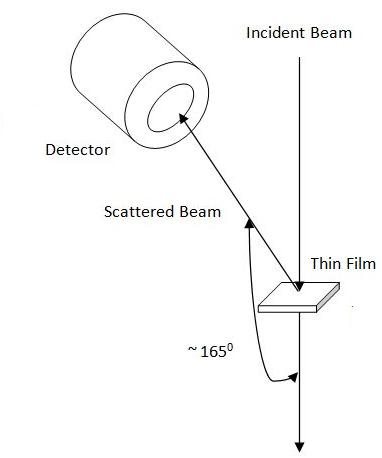# 1.15: Rutherford Backscattering of Thin Films

$$\newcommand{\vecs}{\overset { \rightharpoonup} {\mathbf{#1}} }$$ $$\newcommand{\vecd}{\overset{-\!-\!\rightharpoonup}{\vphantom{a}\smash {#1}}}$$$$\newcommand{\id}{\mathrm{id}}$$ $$\newcommand{\Span}{\mathrm{span}}$$ $$\newcommand{\kernel}{\mathrm{null}\,}$$ $$\newcommand{\range}{\mathrm{range}\,}$$ $$\newcommand{\RealPart}{\mathrm{Re}}$$ $$\newcommand{\ImaginaryPart}{\mathrm{Im}}$$ $$\newcommand{\Argument}{\mathrm{Arg}}$$ $$\newcommand{\norm}{\| #1 \|}$$ $$\newcommand{\inner}{\langle #1, #2 \rangle}$$ $$\newcommand{\Span}{\mathrm{span}}$$ $$\newcommand{\id}{\mathrm{id}}$$ $$\newcommand{\Span}{\mathrm{span}}$$ $$\newcommand{\kernel}{\mathrm{null}\,}$$ $$\newcommand{\range}{\mathrm{range}\,}$$ $$\newcommand{\RealPart}{\mathrm{Re}}$$ $$\newcommand{\ImaginaryPart}{\mathrm{Im}}$$ $$\newcommand{\Argument}{\mathrm{Arg}}$$ $$\newcommand{\norm}{\| #1 \|}$$ $$\newcommand{\inner}{\langle #1, #2 \rangle}$$ $$\newcommand{\Span}{\mathrm{span}}$$$$\newcommand{\AA}{\unicode[.8,0]{x212B}}$$

#### Introduction

One of the main research interests of the semiconductor industry is to improve the performance of semiconducting devices and to construct new materials with reduced size or thickness that have potential application in transistors and microelectronic devices. However, the most significant challenge regarding thin film semiconductor materials is measurement. Properties such as the thickness, composition at the surface, and contamination, all are critical parameters of the thin films. To address these issues, we need an analytical technique which can measure accurately through the depth of the of the semiconductor surface without destruction of the material. Rutherford backscattering spectroscopy is a unique analysis method for this purpose. It can give us information regarding in-depth profiling in a non-destructive manner. However X-ray photo electron spectroscopy (XPS), energy dispersive X-ray analysis (EDX) and Auger electron spectroscopy are also able to study the depth-profile of semiconductor films. Table $$\PageIndex{1}$$ demonstrates the comparison between those techniques with RBS.

 Method Destructive Incident Particle Outgoing Particle Detection Limit Depth Resolution RBS No Ion Ion ~1 10 nm XPS Yes X-ray photon Electron ~0.1-1 ~1 µm EDX Yes Electron X-ray photon ~0.1 1.5 µm Auger Yes Electron Electron ~0.1-1 1.5 nm

## Basic Concept of Rutherford Backscattering Spectroscopy

At a basic level, RBS demonstrates the electrostatic repulsion between high energy incident ions and target nuclei. The specimen under study is bombarded with monoenergetic beam of 4He+ particles and the backscattered particles are detected by the detector-analysis system which measures the energies of the particles. During the collision, energy is transferred from the incident particle to the target specimen atoms; the change in energy of the scattered particle depends on the masses of incoming and target atoms. For an incident particle of mass M1, the energy is E0 while the mass of the target atom is M2. After the collision, the residual energy E of the particle scattered at angle Ø can be expressed as:

$E\ =\ k^{2}E_{0} \label{1}$

$\ k\ =\ \frac{(M_{1}\ cos(\theta )\ +\ \sqrt{M_{2}^{2}\ -\ M_{1}^{2}sin^{2}\theta })}{M_{1}\ +\ M_{2}} \label{2}$

where k is the kinematic scattering factor, which is actually the energy ratio of the particle before and after the collision. Since k depends on the masses of the incident particle and target atom and the scattering angle, the energy of the scattered particle is also determined by these three parameters. A simplified layout of backscattering experiment is shown in Figure $$\PageIndex{1}$$.Figure $$\PageIndex{1}$$ Schematic representation of the experimental setup for Rutherford backscattering analysis.

The probability of a scattering event can be described by the differential scattering cross section of a target atom for scattering an incoming particle through the angle Ø into differential solid angle as follows,

$\frac{d \sigma R}{d \phi }\ = (\frac{zZe2}{2E_{0}sin(2\theta )} )\ =\ \frac{[cos\theta \ +\ \sqrt{1\ -\ (\frac{M_{1}}{M_{2}}sin\theta )^{2}}]^{2}}{\sqrt{1\ -\ (\frac{M_{1}}{M_{2}}sin\theta )^{2}}} \label{3}$

where dσR is the effective differential cross section for the scattering of a particle. The above equation may looks complicated but it conveys the message that the probability of scattering event can be expressed as a function of scattering cross section which is proportional to the zZ when a particle with charge ze approaches the target atom with charge Ze.

Helium ions not scattered at the surface lose energy as they traverse the solid. They lose energy due to interaction with electrons in the target. After collision the He particles lose further energy on their way out to the detector. We need to know two quantities to measure the energy loss, the distance Δt that the particles penetrate into the target and the energy loss ΔE in this distance Figure $$\PageIndex{2}$$. The rate of energy loss or stopping power is a critical component in backscattering experiments as it determines the depth profile in a given experiment.Figure $$\PageIndex{2}$$ Components of energy loss for a ion beam that scatters from depth t. First, incident beam loses energy through interaction with electrons ΔEin. Then energy lost occurs due to scattering Ec. Finally outgoing beam loses energy for interaction with electrons ΔEout. Adapted from L. C. Feldman and J. W. Mayer, Fundamentals of Surface and Thin Film Analysis , North Holland-Elsevier, New York (1986).

In thin film analysis, it is convenient to assume that total energy loss ΔE into depth t is only proportional to t for a given target. This assumption allows a simple derivation of energy loss in backscattering as more complete analysis requires many numerical techniques. In constant dE/dx approximation, total energy loss becomes linearly related to depth t, Figure $$\PageIndex{3}$$.Figure $$\PageIndex{3}$$ Variation of energy loss with the depth of the target in constant dE/dx approximation.

## Experimental Set-up

The apparatus for Rutherford backscattering analysis of thin solid surface typically consist of three components:

1. A source of helium ions.
2. An accelerator to energize the helium ions.
3. A detector to measure the energy of scattered ions.

There are two types of accelerator/ion source available. In single stage accelerator, the He+ source is placed within an insulating gas-filled tank (Figure $$\PageIndex{4}$$). It is difficult to install new ion source when it is exhausted in this type of accelerator. Moreover, it is also difficult to achieve particles with energy much more than 1 MeV since it is difficult to apply high voltages in this type of system.Figure $$\PageIndex{4}$$ Schematic representation of a single stage accelerator.

Another variation is “tandem accelerator.” Here the ion source is at ground and produces negative ion. The positive terminal is located is at the center of the acceleration tube (Figure $$\PageIndex{5}$$). Initially the negative ion is accelerated from ground to terminal. At terminal two-electron stripping process converts the He- to He++. The positive ions are further accelerated toward ground due to columbic repulsion from positive terminal. This arrangement can achieve highly accelerated He++ ions (~ 2.25 MeV) with moderate voltage of 750 kV.Figure $$\PageIndex{5}$$ Schematic representation of a tandem accelerator.

Particles that are backscattered by surface atoms of the bombarded specimen are detected by a surface barrier detector. The surface barrier detector is a thin layer of p-type silicon on the n-type substrate resulting p-n junction. When the scattered ions exchange energy with the electrons on the surface of the detector upon reaching the detector, electrons get promoted from the valence band to the conduction band. Thus, each exchange of energy creates electron-hole pairs. The energy of scattered ions is detected by simply counting the number of electron-hole pairs. The energy resolution of the surface barrier detector in a standard RBS experiment is 12 - 20 keV. The surface barrier detector is generally set between 90° and 170° to the incident beam. Films are usually set normal to the incident beam. A simple layout is shown in Figure $$\PageIndex{6}$$.Figure $$\PageIndex{6}$$ Schematic representation general setup where the surface barrier detector is placed at angle of 165° to the extrapolated incident beam.

## Depth Profile Analysis

As stated earlier, it is a good approximation in thin film analysis that the total energy loss ΔE is proportional to depth t. With this approximation, we can derive the relation between energy width ΔE of the signal from a film of thickness Δt as follows,

$\Delta E\ =\ \Delta t (k\ \frac{dE}{dx_{in}}\ +\ \frac{1}{\cos \theta } \ \frac{dE}{dx_{out}}) \label{4}$

where Ø = lab scattering angle.

It is worth noting that k is the kinematic factor defined in equation above and the subscripts “in” and “out” indicate the energies at which the rate of loss of energy or dE/dx is evaluated. As an example, we consider the backscattering spectrum, at scattering angle 170°, for 2 MeV He++ incidents on silicon layer deposited onto 2 mm thick niobium substrate Figure $$\PageIndex{7}$$.Figure $$\PageIndex{7}$$ The backscattering spectrum for 2.0 MeV He ions incident on a silicon thin film deposited onto a niobium substrate. Adapted from P. D. Stupik, M. M. Donovan, A. R. Barron, T. R. Jervis and M. Nastasi, Thin Solid Films, 1992, 207, 138.

The energy loss rate of incoming He++ or dE/dx along inward path in elemental Si is ≈24.6 eV/Å at 2 MeV and is ≈26 eV/Å for the outgoing particle at 1.12 MeV (Since K of Si is 0.56 when the scattering angle is 170°, energy of the outgoing particle would be equal to 2 x 0.56 or 1.12 MeV) . Again the value of ΔESi is ≈133.3 keV. Putting the values into above equation we get

$\Delta t \approx \frac{133.6\ keV}{(0.56*24.6\ \frac{eV}{Å}) \ +\ (\frac{1}{\cos 170^{\circ } }\ *\ 26\ \frac{eV}{Å})} \nonumber$

$=\ \frac{133.3\ keV}{13.77\ eV/Å \ +\ 29.985\ eV/Å} \nonumber$

$=\ \frac{133.3\ keV}{40.17 eV/Å} \nonumber$

$=\ 3318\ Å \nonumber$

Hence a Si layer of ca. 3300 Å thickness has been deposited on the niobium substrate. However we need to remember that the value of dE/dx is approximated in this calculation.

## Quantitative Analysis

In addition to depth profile analysis, we can study the composition of an element quantitatively by backscattering spectroscopy. The basic equation for quantitative analysis is

$Y\ =\ \sigma \Omega Q N \Delta t \nonumber$

Where Y is the yield of scattered ions from a thin layer of thickness Δt, Q is the number of incident ions and Ω is the detector solid angle, and NΔt is the number of specimen atoms (atom/cm2). Figure $$\PageIndex{8}$$ shows the RBS spectrum for a sample of silicon deposited on a niobium substrate and subjected to laser mixing. The Nb has reacted with the silicon to form a NbSi2 interphase layer. The Nb signal has broadened after the reaction as show in Figure $$\PageIndex{8}$$.

We can use ratio of the heights HSi/HNb of the backscattering spectrum after formation of NbSi2 to determine the composition of the silicide layer. The stoichiometric ratio of Nb and Si can be approximated as,

$\frac{N_{Si}}{N_{Nb}}\ \approx \frac{[H_{Si}\ *\ \sigma_{Si}]}{[H_{Nb}\ *\ \sigma _{Nb}]} \nonumber$

Hence the concentration of Si and Nb can be determined if we can know the appropriate cross sections σSiand σNb. However the yield in the backscattering spectra is better represented as the product of signal height and the energy width ΔE. Thus stoichiometric ratio can be better approximated as

$\frac{N_{Si}}{N_{Nb}}\ \approx \frac{[H_{Si}\ *\ \Delta E_{Si}\ *\ \sigma_{Si}]}{[H_{Nb}\ *\ \Delta E_{Nb}\ *\ \ \sigma _{Nb}]} \nonumber$

## Limitations

It is of interest to understand the limitations of the backscattering technique in terms of the comparison with other thin film analysis technique such as AES, XPS and SIMS (Table $$\PageIndex{1}$$). AES has better mass resolution, lateral resolution and depth resolution than RBS. But AES suffers from sputtering artifacts. Compared to RBS, SIMS has better sensitivity. RBS does not provide any chemical bonding information which we can get from XPS. Again, sputtering artifact problems are also associated in XPS. The strength of RBS lies in quantitative analysis. However, conventional RBS systems cannot analyze ultrathin films since the depth resolution is only about 10 nm using surface barrier detector.

## Summary

Rutherford Backscattering analysis is a straightforward technique to determine the thickness and composition of thin films (< 4000 Å). Areas that have been lately explored are the use of backscattering technique in composition determination of new superconductor oxides; analysis of lattice mismatched epitaxial layers, and as a probe of thin film morphology and surface clustering.

This page titled 1.15: Rutherford Backscattering of Thin Films is shared under a CC BY 4.0 license and was authored, remixed, and/or curated by Pavan M. V. Raja & Andrew R. Barron (OpenStax CNX) via source content that was edited to the style and standards of the LibreTexts platform; a detailed edit history is available upon request.